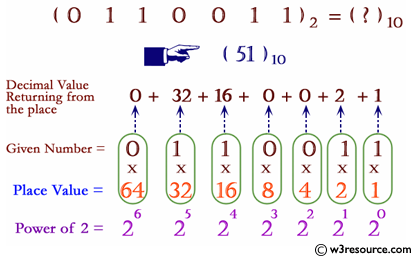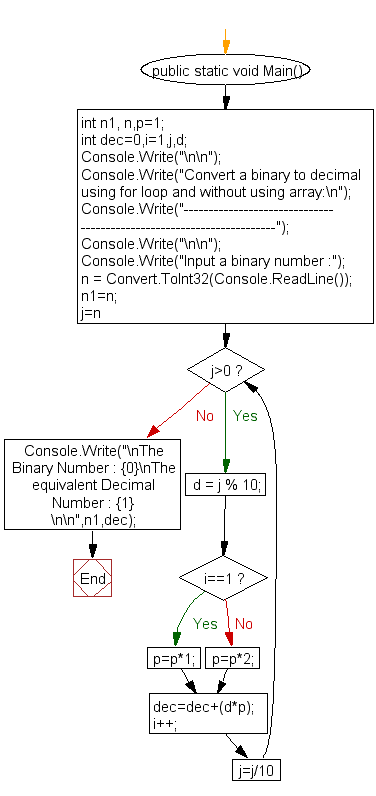﻿ C# - Without an array, convert a binary to a decimal# C# Sharp Exercises: Convert a binary to a decimal using for loop and without using an array

## C# Sharp For Loop: Exercise-42 with Solution

Write a program in C# Sharp to convert a binary number into a decimal number without using an array, function and while loop.Sample Solution:-

C# Sharp Code:

``````using System;
public class Exercise42
{
public static void Main()
{       int n1, n,p=1;
int dec=0,i=1,j,d;
Console.Write("\n\n");
Console.Write("Convert a binary to decimal using for loop and without using array:\n");
Console.Write("---------------------------------------------------------------------");
Console.Write("\n\n");

Console.Write("Input a binary number :");
n1=n;
for (j=n;j>0;j=j/10)
{
d = j % 10;
if(i==1)
p*=1;
else
p*=2;

dec=dec+(d*p);
i++;
}
Console.Write("\nThe Binary Number : {0}\nThe equivalent Decimal  Number : {1} \n\n",n1,dec);
}
}
```
```

Sample Output:

```Convert a binary to decimal using for loop and without using array:
---------------------------------------------------------------------
Input a binary number :1000001
The Binary Number : 1000001
The equivalent Decimal  Number : 65
```

Flowchart:C# Sharp Code Editor:

Contribute your code and comments through Disqus.

What is the difficulty level of this exercise?

Test your Programming skills with w3resource's quiz.

﻿